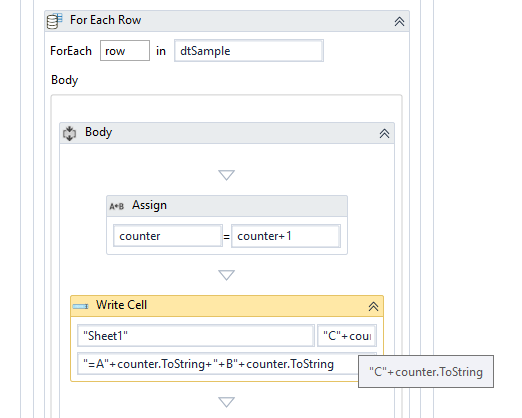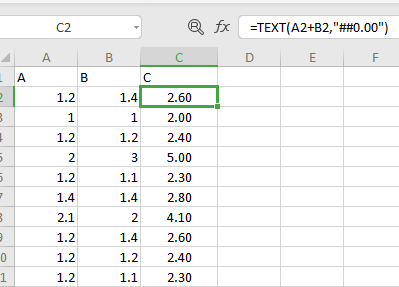# How to set formula in a column in excel

Here I have a data table with 3 columns A,B,C. value of column C = value of A + value of B. how to set formula and need to write this data table to excel

@rifnanahas

Check this out !!
Flow.zip (19.2 KB)

1 Like

But I need to write the formula in column C

just run the workflow it will write in Column C itself

I will attach a screenshot of what I expect

Here in C2 how it appears likewise I need in full cells in Column C

Just loop through the data table and use Write Cell to write the formula in column C.
Use counter to identify the row and place the correct formula in the target cell.
counter should be in the workflow scope and with 1 as the initial value.Output:1. Kindly use write cell in C1 alone and write the formula c=A+B
2. Then Read entire excel sheet and name as dt
3. Copy the C1 Formula using copy activity
4. use select Area activity it will be inside excel Application scope and put in the select area activity “C1:C”+dt.Rows.Count.Tostring .
5. Now paste the value it will paste in the selected region and at last put saveworkbook activity and close it.
1 Like

column value is a decimal number. I need to round the value to only 2 decimal points.
How to round the value inside the write cell?

use this function “math.Round(decimalvalue,2)” in linq to roun up all the decimal values in the column or you can loop and do .

Use this excel formula in the cell value. 2 is the decimal place. Change it to any number depending on the decimal place you need for the results.

“=ROUND”+“(A”+counter.ToString+“+B”+counter.ToString+“,2)”You can also use…
“=TEXT”+“(A”+counter.ToString+“+B”+counter.ToString+“,”“##0.00"”)"